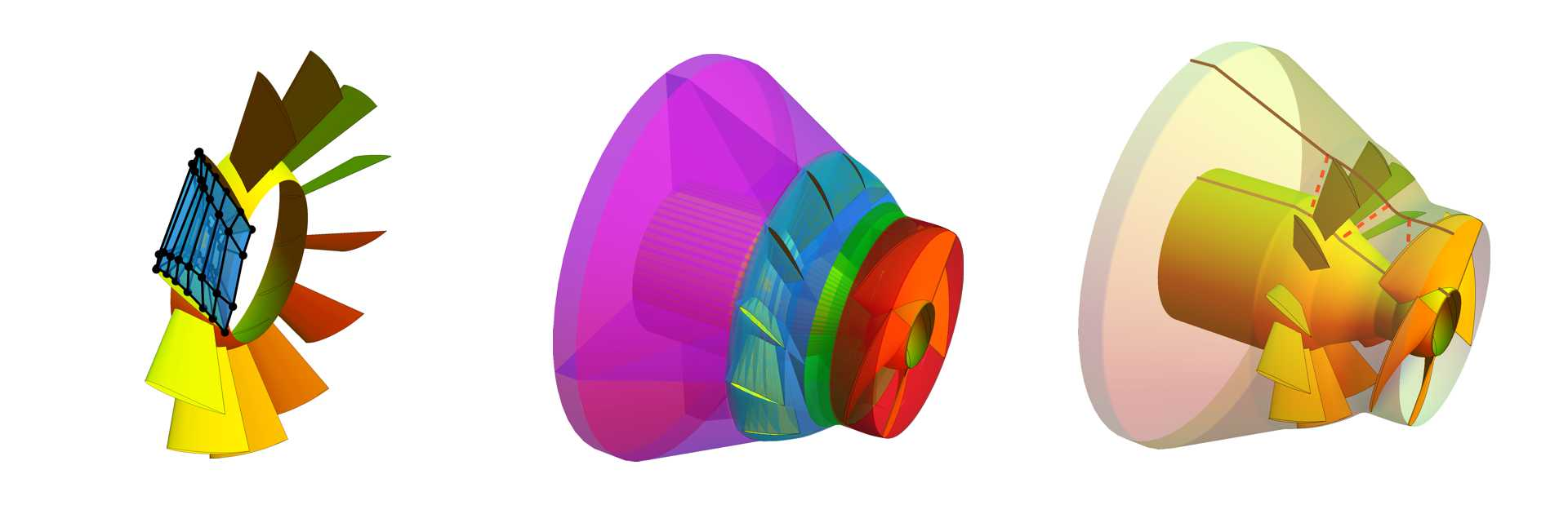# Mathematical Modelling

Study of dynamic systems, modelling by using non-linear differential equations, numeric and geometric modelling and simulations, structures and methods of discrete mathematics.

The main objective is to provide theoretical support to the other activities involved in this project. On the other hand, independent research in mathematics is also included in the activity as an important ingredient. Consequently, the second (and equally important) objective is to develop advanced mathematical methods and tools and apply them to obtain descriptions of those selected problems and phenomena of the real world that can be modelled using continuous and discrete mathematical structures. A strong emphasis is put on the questions of solvability, stability and robustness of the methods being developed. Current efforts in many disciplines of science are aimed at obtaining more accurate descriptions of the phenomena being studied. This often requires taking an approach that is radically different from those applied until recently, and using different tools (for instance, passing from linear models to non-linear ones). From the mathematical viewpoint, these are fundamental changes requiring a completely different approach both to the description of the problem and to its subsequent solution and algorithmisation. This brings the need to constantly work on an advanced, highly applicable theoretical apparatus, and at the same time to look for ways to use and implement previously known results in new areas.